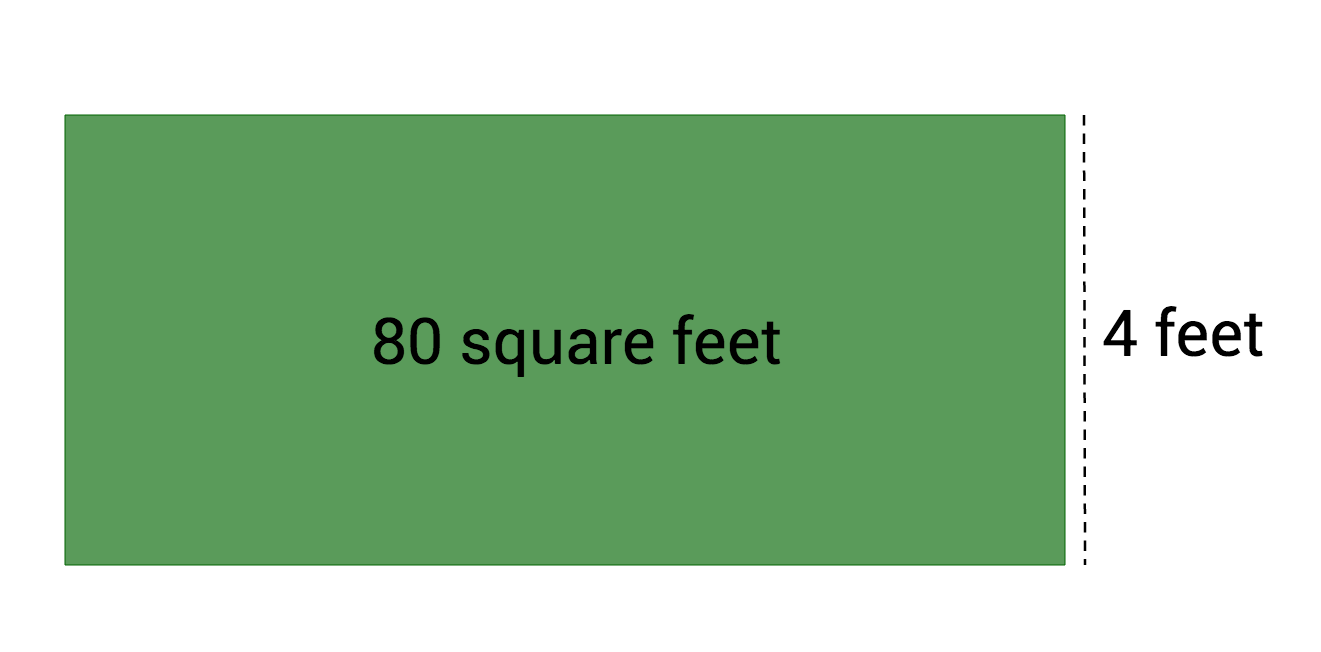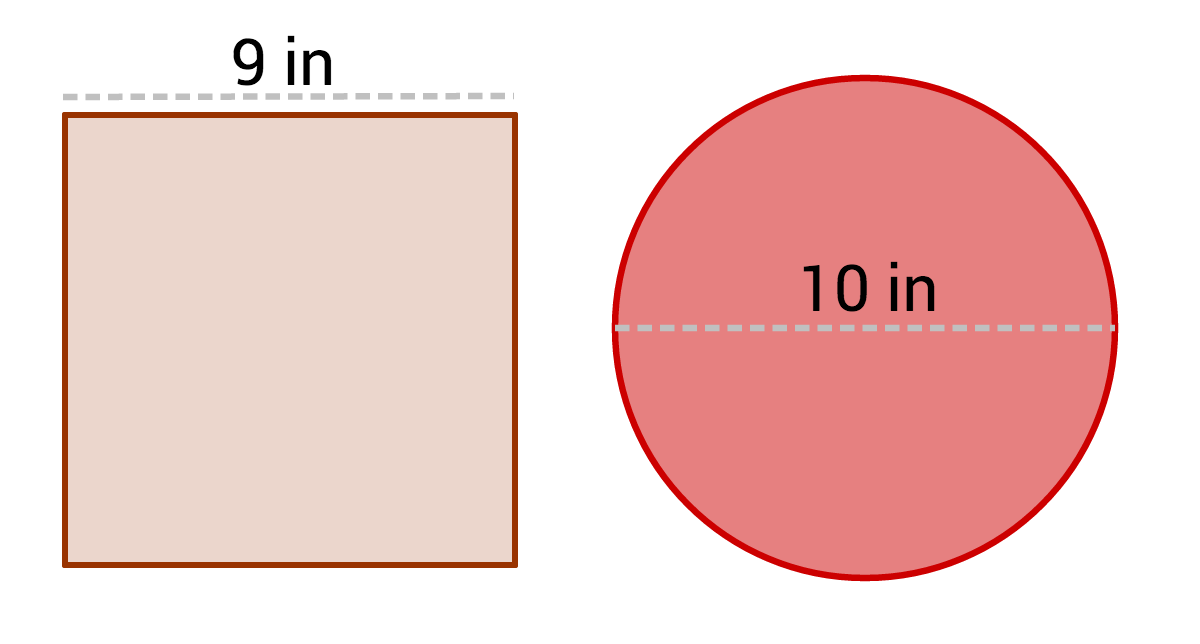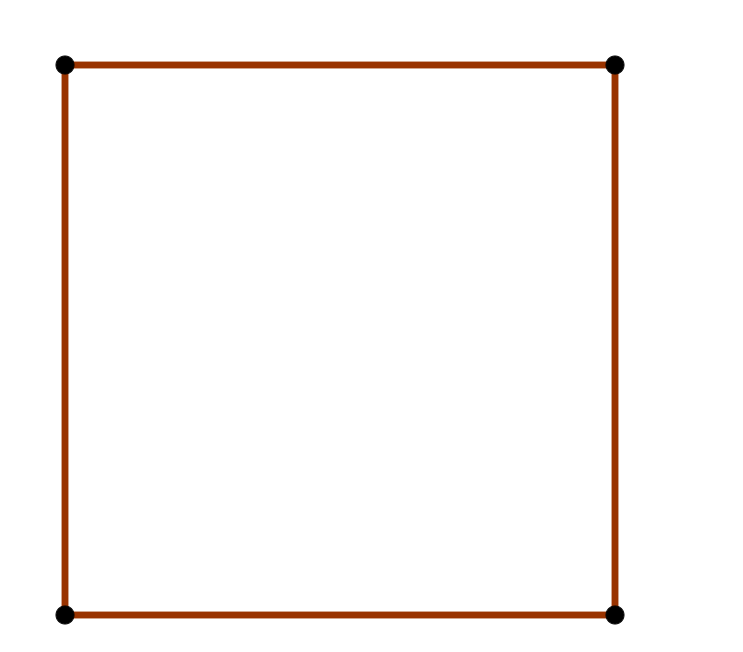Geometry

Area ApplicationsA rectangular garden covers 80 square feet. If it is 4 feet wide, how long is it?

Suppose that you need a triangle which has an area of $20 \text{ cm}^2$ and a base of 10 cm. Is there more than one possible triangle with these characteristics?If the pizzas cost the same amount, which pizza is a better deal?

A: The 9 inch square pizza.

B: The 10 inch round pizza.If every rectangle has the same perimeter, which one has the greatest area?Four toothpicks create the square shown. You need to quadruple the area using the minimum number of toothpicks. How many toothpicks will the new figure have?

×